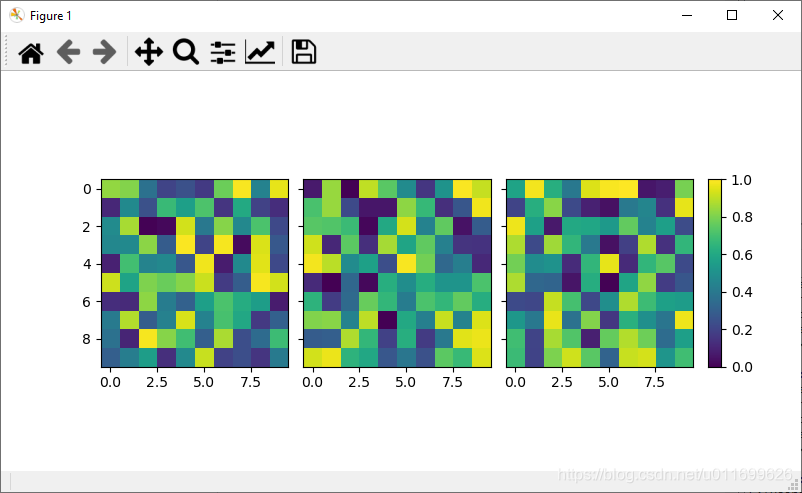# matplotlib 多个图像共用一个colorbar的实现示例

2020-09-15 04:08 85 查看

```# -*- coding: utf-8 -*-
"""
Created on Sat Sep 5 18:05:11 2020
@author: 15025
draw three figures with one common colorbar
"""

import numpy as np
import matplotlib.pyplot as plt
from mpl_toolkits.axes_grid1 import ImageGrid

class Visualazation:
def mainProgram(self):
# Set up figure and image grid
fig = plt.figure(figsize=(8, 4))

grid = ImageGrid(fig, 111,
nrows_ncols=(1,3),
share_all=True,
cbar_location="right",
cbar_mode="single",
cbar_size="7%",
)

# Add data to image grid
for ax in grid:
im = ax.imshow(np.random.random((10,10)), vmin=0, vmax=1)

# Colorbar
ax.cax.colorbar(im)
ax.cax.toggle_label(True)

plt.show()

if __name__ == "__main__":
main = Visualazation()
main.mainProgram()````ImageGrid()`函数参数说明：`nrows_ncols=(1,3)`表示创建一个`1``3`列的画布。`share_all=True`表示所画的图像公用x坐标轴和y坐标轴。`cbar_location="right"`表示`colorbar`位于图像的右侧，当然也可以位于上方，下方和左侧。`cbar_mode="single"`表示三个图像公用一个`colorbar``cbar_size="7%"`表示`colorbar`的尺寸，默认值为`5%``cbar_pad=0.15`表示图像与`colorbar`之间的填充间距，默认值为`5%`。可以自行调整以上数值进行尝试。